﻿ Heat Mass Transfer of Nanofluids Flow with Soret Effect In the Presence of Thermal Radiation

# Journal of Physical MathematicsOpen Access

All submissions of the EM system will be redirected to Online Manuscript Submission System. Authors are requested to submit articles directly to Online Manuscript Submission System of respective journal.
• Research Article
• J Phys Math 2019, Vol 10(3): 306

# Heat Mass Transfer of Nanofluids Flow with Soret Effect In the Presence of Thermal Radiation

Zayyanu SY*, Hamza MM and Uwanta IJ
Department of Mathematics, Usmanu Danfodiyo University, Sokoto, Nigeria
*Corresponding Author: Zayyanu SY, Department of Mathematics, Usmanu Danfodiyo University, Sokoto, Nigeria, Tel: 07032968681, Email: [email protected]

Received Date: Aug 06, 2019 / Accepted Date: Sep 25, 2019 / Published Date: Oct 08, 2019

### Abstract

This study investigates the Soret effects on Natural convection flow of Nano fluids over an infinite vertical plate, in the presence of thermal radiation and applied magnetic field. The fluid is assumed to be electrically conducting water based Cu-nanofluid. The Tiwari and Das model is used to model the Nano fluid, whereas Rosseland approximation is used for thermal radiation effect. Solutions for time dependent velocity, temperature and concentration equations are obtained using Laplace transform technique. The physical quantities of engineering interest such as skin friction and Sherwood number are also computed. The obtained analytical solutions satisfy all imposed initial and boundary conditions. The effect of various parameters controlling the physical situation is discussed with the aid of line graphs. During the course of computation, excellent result was found from various physical parameters embedded in the problem.

Keywords: Nanofluid; Soret effect; Nanoparticle; Thermal radiation; Heat transfer; Mass transfer

#### Nomenclature

C: Dimensionless Concentration; Cf Skin friction coefficient; Cp: Specific heat capacity of fluid (J/KgK); g: Acceleration due to gravity; Kr: Chemical reaction parameter; Sr: Soret number; K: Thermal conductivity; K*: Rosseland mean absorption coefficient; M: Magnetic parameter; Nu: Nusselt number; Pr: Prandtl number; Q₀: Chemical absorption coefficient; Grc: Solutal grashof number; Grt: Thermal grashof number; qr: Radiative heat flux; R: Radiation parameter; Sc: Schmidt number; Sh: Sherwood number; t: Dimensionless time; T: Dimensional temperature; θ: Dimensionless temperature; u*: Dimensional velocity; u: Dimensionless velocity; t*: Dimensional time; y*: Cartesian coordinate along the plate; Dnf: Mass diffusion coefficient; K*o: Chemical reaction coefficient

#### Greek Symbols

ν: Kinematic viscosity; μnf : Dynamic viscosity (kg/m-1s-1); ϕ: Nanoparticle volume fraction; σ*: Stefan-Boltzmann constant; σ: Electric conductivity of fluid; ρ: Density; β: Thermal expansion coefficient; p,q: Laplace parameters

#### Subscript

f: Based fluid; s: Solid nanoparticle; w: At the wall condition; ∞: Far from the wall

#### Introduction

Recent advances in nanotechnology have allowed the development of a new category of fluids termed nanofluids. A nanofluid refers to the suspension of nanosize particles, which are suspended in the based fluid with low thermal conductivity, these particles also called Nanoparticles, have a diameter of less than 100 nm. The base fluid or dispersing medium can be aqueous or non-aqueous in nature.

Nanofluids or so called smart fluids research has attracted considerable attention in recent years owing to their significance in engineering application and technology. The broad range of current and future applications has been given in the studies by, Wang and Mujumdar , Kakac and Pramuanjaroenkij , Eapen  and Fan and Wang .

Recently, Sheikholeslami and gamji  studied the MHD effects on Nanofluid flow in a permeable channel. Kuznetsov and Nield  critically analyzed the natural convection flow of a Nanofluid past a vertical plate. The problem of transient MHD free convection flow of a Nanofluid over a rotating vertical plate is addressed by Hamad and Pop . They solved the governing equations analytically using the perturbation method and found that, the inclusion of nanoparticles into the based fluid is capable of varying the flow pattern. Turkyilmazoglu  reported the exact solution for heat and mass transfer of magneto hydrodynamic flow of nanofluid. Further, Turkyilmazoglu and Pop  have examined the heat and mass transfer of unsteady natural convection flow of nanofluid past a vertical plate with thermal radiation. Das  discussed the problem of free convection flow of nanofluid bounded by moving vertical plate with constant heat source and convective boundary condition in a rotating frame of reference. He found that, the skin friction coefficient increases with an increase in nanoparticle volume fraction. However, Sheikholeslami and Gamji  have presented the heat and mass transfer behavior of unsteady flow of Nanofluid between parallel plates in the presence of thermal radiation. They concluded that, the solutal boundary layer thickness increases with increase in radiation parameter. Giresha  have studied the effect of nanoparticles on flow and heat transfer of dusty fluid. Das and Jana  studied the MHD flow past a moving vertical plate in the presence of thermal radiation using Laplace transform method for closed form expressions of flow fields, they found that, the sheer stress at the plate for Cu-water nanofluid is found to be lower. Heat transfer in flow of nanofluid with heat source/sink and an induced magnetic field is addressed by Giresha . The boundary layer in Newtonian and porous media filled by nanofluids over fixed and moving boundaries have been studied by Mahdy and Elshehabey .

In many industrial and engineering applications, the heat and mass transfer is a consequence of buoyancy caused by thermal diffusion and chemical species, therefore, the study of heat and mass transfer is needed for improving many technologies such as polymer and ceramic production, food processing, gas production, geothermal reservoirs, thermal insulations and so on. The heat and mass transfer flow of nanofluids in the presence of magnetic field also find a variety of applications such as energy saving, reduction of production time and also a greater quality of the final product. Heat and mass transfer under the influence of chemical reaction has also attracted considerable attention of many authors due to wide range of applications. In view of these, conjugate heat and mass transfer flow past a vertical permeable plate with thermal radiation and chemical reaction is investigated by Pal and Talukdar . They found that, the thermal radiation and chemical reaction effect decrease the velocity and concentration profiles. Numerical investigation of heat and mass transfer of an electrically conducting fluid over a moving surface with first order chemical reaction is carried out by Chamkha  Uwanta and Omokhuale  have analyzed the influence of radiation and chemical reaction effect on heat and mass transfer of a viscoelastic fluid in a mixed plane. Later, the combined effects of radiation and chemical reaction on the MHD free convection flow of an electrically conducting viscous fluid over an inclined plate have been studied by Ali  and have obtained a closed form solution by employing laplace transform technique. Mahanthesh  studied Heat and mass transfer effects on the mixed convective flow of chemically reacting nanofluid past a moving/stationary vertical plate. Recently, Fetecau , studied natural convection flow of fractional nanofluids over an isothermal vertical plate.

On the other hand, Motivated by aforementioned studies, we intend to investigate/extend the study reported by Mahanthesh , the effect of heat and mass transfer on natural convection flow over a vertical plate, the Soret effects, chemical reaction are also considered, to best of our knowledge, this study has not been considered by any author, our interest here, is to obtain the closed form solution for the present problem by employing Laplace Transform technique, the basic partial differential equations are derived by using Boussinesq approximation and then are transformed to dimensionless equations using suitable non-dimensional parameters. The resultant equations have been solved analytically.

#### Mathematical Formulation and Solution

Consider the natural convection flow, heat and mass transfer of nanofluid, past an infinite vertical plate situated in the (x*,z*)-plane of a fixed Cartesian coordinate system Ox*y*z*. The plate is at rest initially with constant ambient temperature and concentration T∞ and C∞ respectively, uniform transverse magnetic field of strength (β0) and radiative heat flux (qr) are applied perpendicular to the plate. It is assumed that the pressure gradient is neglected and the fluid is electrically conducting, water based nanofluid embedded with copper nanoparticles. Since the plate is infinite, all of the physical quantities describing the fluid motion are functions of y* and t*.

The thermo physical properties of copper (Cu) are given in Table 1 (Oztop and Abu-nada ).

Physical properties Base fluid Cu
ρ (kg/m3) 997.1 8933
Cρ (J/kgK) 4179 385
K (W/mK) 0.613 401
βt × 105 (k-1) 21 1.67
βc × 106 (m2/h) 298.2 3.05
ϕ 0.0 0.05
σ (s/m) 5.5 × 10-6 59.6 × 106

Table 1: Thermo physical properties of copper Cu.

Assuming small differences between the temperature T (y*,t*) and the stream temperature T∞ and adopting the Rosseland approximation , the radiative heat flux qr (y*,t*) can be linearized to: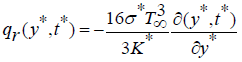(1)

Where σ* is the Stefan-Boltzmann constant and k* is the mean absorption coefficient. In view of the above assumption and taking into account the nanofluid model proposed by Tiwari and Das , the governing equations can be written as: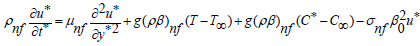(2)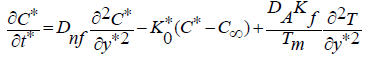(3)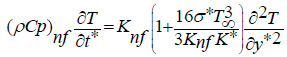(4)

In the above equations, u* (y*,t*) is the fluid velocity in the x* direction, Tw is constant plate temperature (Tw>T) or (Tw<T) corresponds to the Heated or cooled plate respectively), g is the acceleration due to gravity, σnf is the effective thermal conductivity, K0* the chemical reaction coefficient, Dnf is the diffusion coefficient, Tm is the mean absorption, ρnf is the density of the nanofluid, μnf is the dynamic viscosity of the nanofluid, βnf is the thermal expansion coefficient of the nanofluid. The effective properties of nanofluid are given by the following co-relations :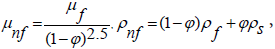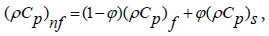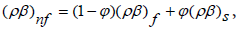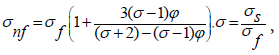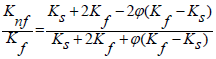(5)

Where ϕ the volume fraction of nanoparticles, ρf and ρs are density of base fluid and nanoparticles respectively, Cpf and Cps are specific heat of the base fluid and nanoparticles respectively, σf and σs are electrical conductivity of base fluid and nanoparticles correspondingly, βf and βs are thermal expansion coefficient of the base fluid and nanoparticles respectively, Kf and Ks are thermal conductivity of base fluid and nanoparticles.

If no slipping exists between the fluid and the plate, the appropriate initial and boundary conditions are: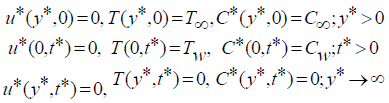(6)

The non-dimensional quantities introduced in the above equations are defined as: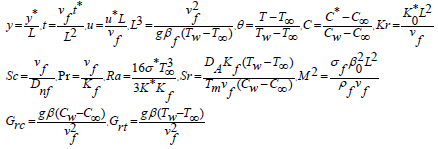(7)

On substituting these dimensionless variables into equations (2), (3) and (4), one can get ;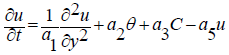(8)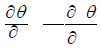(9)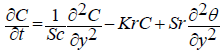(10)

With initial and boundary conditions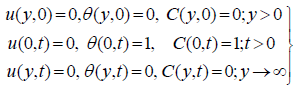(11)

By applying the Laplace Transform on both sides of equations (8)- (10), we have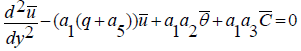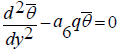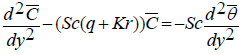With corresponding initial and boundary conditions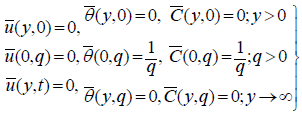(15)

Where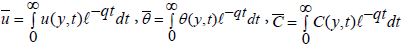and q > 0 . Now by solving the above system, one can have;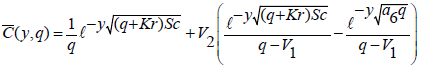(16)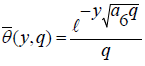(17)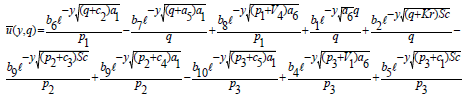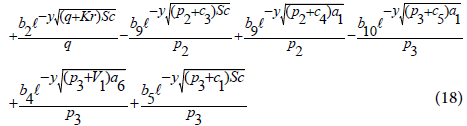In order to determine, the flow fields in the time domain, by applying Laplace Inverse Transform on both sides of equations (16)- (18), we get;

Velocity equation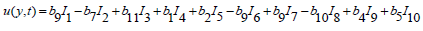(19)

Temperature equation

θ (y,t)= I4 (20)

Concentration equation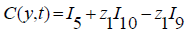(21)

In order to determine the three entities of physical interest, namely the skin friction coefficient (Cf), Nusselt number (Nu) and Sherwood number (Sh), we use the relations;

Skin Friction (Cf)

The Skin friction coefficient at the plate y=0 is given by;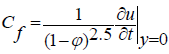(22)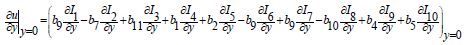Nusselt number (Nu)

The Nusselt number (Nu) at the plate y=0 is given by;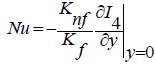(23)

Sherwood number (Sh)

The Sherwood number (Sh) at the plate y=0 is given by;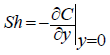(24)

Where: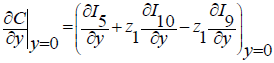#### Results and Discussion

In the present study, the Laplace Transform technique (LT) has been employed to obtain the analytical expressions for Velocity, Temperature and Concentration profiles as well the Skin friction coefficient, Nusselt number and Sherwood number. In order to have the clear insight of the problem, a numerical computation has been carried out for different values of pertinent parameters. Throughout our numerical computation, the value of Prandtl number (Pr ) has been taken to be 6.2 which corresponds to water, Radiation parameter (R) has been taken as 1 , Schmidt number (Sc) is taken to be 0.6 which correspond to water vapor, other parameters are varied over the range which are listed in the figures legend (Figure 1).

Figure 2 is prepared to show that, an increase in chemical reaction parameter (Kr) decreases the concentration profile and vice-versa. The reason behind this is that, the number of solute molecules undergoing chemical reactions get increased as chemical reaction parameter increases, which lead to decrease in concentration profile. While Figure 3 shows that as the Soret number (Sr) increases, the solutal concentration boundary layer thickness of nanofluid also increases. Figure 4 highlighted the effect of Soret parameter on velocity when other parameters are considered as constants. It is noticed that increasing the value of Soret parameter the velocity profile in the boundary layer increases and vice versa. In Figure 5, depicts the effects of Solutal grashof number, it is described as the ratio of the species buoyancy force to the viscous hydrodynamic force. As solutal grashof number increases, the viscous hydrodynamic force decreases, as a result, the momentum boundary layer thickens and vice-versa. Figure 6 is prepared to show the effect of Magnetic parameter, on velocity profile, it is observed that the fluid velocity decreases as the magnetic field parameter increases, this is due to the fact that, the application of transverse magnetic field to an electrically conducting fluid gives rise to resistive type of force called Lorentz force. This force has the tendency to slow down the motion of the fluid, consequently, the fluid velocity reduced significantly .

Figure 2: Effects of chemical reaction parameter (Kr) on concentration profile when Sc=0.6, t=0.5.

Figure 4: Effects of Soret number (Sr) on velocity profile when t=0.5, R-1, ϕ=0.2, Pr=6.2, Sc=0.6, M=0.1, Grc=1, Grt=0.1, Kr=5.

Figure 5: Effects of Solutal grashof number (Grc) on velocity profile when Kr=5, t=0.5, R-1, ϕ=0.2, Pr=6.2, Sc=0.6, M=0.1, Sr=1, Grt=0.1.

Figure 6: Effects of Magnetic parameter (M) on velocity profile when Kr=5, t=0.5, R-1, ϕ=0.2, Pr=6.2, Sc=0.6, Sr=1, Grc=1, Grt=0.1.

Figure 7 highlighted the effects of Soret number on Sherwood number against Chemical reaction parameter, it is noted that increasing soret number will increase the Sherwood number significantly.

Figure 8 delicates the effects of Soret number on Skin Friction, it is observed that increasing Soret number decrease the Skin friction coefficient and vice versa. Figure 9 delicates the effect of magnetic parameter on the skin friction coefficient, it is observed that increase in magnetic parameter decrease the skin friction coefficient and viceversa.

Figure 8: Effects of Soret number (Sr) on Skin friction when Kr=5, t=0.5, R=1, Pr=6.2, Sc=0.6, Grc=1, Grt=0.1, ϕ-0.2.

Figure 9: Effects of Magnetic parameter (M) on Skin friction profile when Kr=5, t=0.5, R=1, Pr=6.2, Sc=0.6, Sr=1, Grc=1, Grt=0.1.

Also Figure 10, shows the effect Solutal grashof number on skin frition coefficient, it is seen that increasing the value of solutal grashof number, the skin friction decreases and vice versa. Figure 11 delincates the effect of thermal grashof number on skin friction, is seen that increasing the value of thermal grashof number, the skin friction increases and vice versa.

Figure 10: Effects of solute grash of number (Grc) on Skin friction profile when Kr=5, t=0.5, R=1, Pr=6.2, Sc=0.6, Sr=1, M=0.1, Grt=0.1.

Figure 11: Effects of solute grashof number (Grc) on Skin friction profile when Kr=5, t=0.5, R=1, Pr=6.2, Sc=0.6, Sr=1, M=0.1, Grc=1.

#### Conclusion

The heat mass transfer of nanofluids flow with Soret effect in the presence of thermal radiation has been studied, the set of governing equations has been solved analytically using Laplace transform technique, the effects of pertinent parameters on the nanofluid concentration, temperature, velocity, rate of mass transfer, rate of heat transfer and skin friction are investigated and analyzed with the help of their graphical representations. Based on the results, the following conclusions are drawn:

• The presence of Soret number increases the concentration and velocity profiles of the fluid.

• Intensifying magnetic field strength stabilizes the velocity of the fluid.

• The influence of solutal grashof number and thermal grashof number are opposite on velocity field.

• As a result of chemical reaction, the rate of the flow enhances.

• An increase in chemical reaction parameter decreases the rate of heat transfer of the fluid.

#### References

Citation: Zayyanu SY, Hamza MM, Uwanta IJ (2019) Heat Mass Transfer of Nanofluids Flow with Soret Effect In the Presence of Thermal Radiation. J Phys Math 10: 306.

Copyright: © 2019 Zayyanu SY, et al. This is an open-access article distributed under the terms of the Creative Commons Attribution License, which permits unrestricted use, distribution, and reproduction in any medium, provided the original author and source are credited.

Select your language of interest to view the total content in your interested language

##### Recommended Journals
Viewmore
###### Article Usage
• Total views: 359
• [From(publication date): 0-0 - Nov 21, 2019]
• Breakdown by view type
• HTML page views: 334Can't read the image? click here to refresh